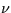Next: 12.9 High-Dispersion Merged Extracted Up: 12 Final Archive Data Previous: 12.7 High-Dispersion Resampled Image

# 12.8 Low-Dispersion Merged Extracted Image FITS File (MXLO)

The data extracted from the low-dispersion SI are stored in the MXLO using a binary table extension with fixed-length floating point vectors to contain the extracted fluxes and associatedflags. Since no primary data are included, the extension header immediately follows the primary header. Each row of the binary table includes the following columns:

• Aperture designation as LARGE' or SMALL', stored in 5 ASCII characters.
• Number of extracted points, one 16-bit integer. The number of extracted points is always 640.
• Starting wavelength, one single-precision floating point value.
• Wavelength increment, one single-precision floating point value.
• Net flux spectrum, array with 640 single-precision floating point values.
• Background flux spectrum, array with 640 single-precision floating point values.
• Sigma vector, array with 640 single-precision floating point values.
•flags, array of 640 16-bit integers stored in two's complement form.
• Absolutely-calibrated net flux spectrum, array with 640 single-precision floating point values.
Wavelengths are linearly sampled to a uniform step size and measured in vacuum. Double aperture low-dispersion spectra will contain two rows in the above format, with one row for each aperture (LARGE first, then SMALL). Note the NAXIS1 keyword in the Binary table extension defines the number of bytes per row in the table and is equal to 15+18*640, or 11,535 bytes.

The absolute calibration covers the range of 1150-1980Å for short-wavelength spectra and 1850-3350Å for long-wavelength spectra. Since the NEWSIPS software extracts data over a slightly larger wavelength range, data points outside this wavelength range are set to 0 in the absolutely-calibrated flux vector and -1 in the sigma vector. The net and background vectors are not affected. The uncalibrated data points are also flagged in theflag vector with the value of -2. Table 12.10 shows the basic FITS Keywords for the MXLO.

Table 12.10:  MXLO - Basic FITS Keywords
Keyword and value Description
 SIMPLE = T Standard FITS Format
 BITPIX = 8 8 bits ASCII
 NAXIS = 0 No image data
 EXTEND = T Extensions are present
 TELESCOP= 'IUE '  International Ultraviolet Explorer
 DATE = 'dd/mm/yy'  Date file is written
 ORIGIN = 'VILSPA '  Institution generating the file
 XTENSION= 'BINTABLE'  Table extension
 BITPIX = 8 Binary data
 NAXIS = 2 Two-dimensional table array
 NAXIS1 = 11535 Bytes per row (15+18*NPOINTS)
 NAXIS2 = n Number of apertures (1-single, 2-both)
 PCOUNT = 0 Number of bytes following data matrix
 GCOUNT = 1 Only one group
 TFIELDS = 9 Number of columns in the table
 TFORM1 = '5A '  Count and data type of field 1
 TTYPE1 = 'APERTURE'  Aperture type (large or small)
 TUNIT1 = ' '  Unitless
 TFORM2 = '1I '  Field 2 has one 2-byte integer
 TTYPE2 = 'NPOINTS '  Number of points
 TUNIT2 = ' '  Unitless
 TFORM3 = '1E '  Count and data type of field 3
 TTYPE3 = 'WAVELENGTH'  3rd field is starting wavelength
 TUNIT3 = 'ANGSTROM'  Unit is angstrom
 TFORM4 = '1E '  Count and data type of field 4
 TTYPE4 = 'DELTAW '  4th field is wavelength increment
 TUNIT4 = 'ANGSTROM'  Unit is Angstrom
 TFORM5 = '640E '  Count and data type of field 5
 TTYPE5 = 'NET '  5th field is net flux array
 TUNIT5 = 'FN '  Unit is IUE FN
 TFORM6 = '640E '  Count and data type of field 6
 TTYPE6 = 'BACKGROUND'  6th field is background flux array
 TUNIT6 = 'FN '  Unit is IUE FN
 TFORM7 = '640E '  Count and data type of field 7
 TTYPE7 = 'SIGMA '  7th field is the sigma
 TUNIT7 = 'ERG/CM2/S/A'  Unit is erg/cm2/sec/Angstrom
 TFORM8 = '640I '  Count and data type of field 8
 TTYPE8 = 'QUALITY '  8th field is the data quality flag
 TUNIT8 = ' '  Unitless
 TFORM9 = '640E '  Count and data type of field 9
 TTYPE9 = 'FLUX '  9th field is the calibrated flux
 TUNIT9 = 'ERG/CM2/S/A'  Unit is erg/cm2/sec/Angstrom
 FILENAME= 'AAAnnnnn.MXLO'  Filename (camera)(number).MXLO
 EXTNAME = 'MXLO '  Name of tableNext: 12.9 High-Dispersion Merged Extracted Up: 12 Final Archive Data Previous: 12.7 High-Dispersion Resampled Image
Karen Levay
12/4/1997